Energy Dissipated by an Aster Accelerated in a Gravitational Field: Estimation of the Lifetime of a Planet or a Star Being Destroyed | OMICS International
Journal of Astrophysics & Aerospace Technology

# Energy Dissipated by an Aster Accelerated in a Gravitational Field: Estimation of the Lifetime of a Planet or a Star Being Destroyed

 Sakho I* Department of Physics, UFR of Sciences and Technologies, University Assane Seck of Ziguinchor, Ziguinchor, Senegal *Corresponding Author : Sakho I Department of Physics UFR of Sciences and Technologies University Assane Seck of Ziguinchor Ziguinchor, Senegal Tel: 04 73 40 72 00 E-mail: [email protected] Received: April 06, 2016 Accepted: April 26, 2015 Published: April 29, 2016 Citation: Sakho I (2016) Energy Dissipated by an Aster Accelerated in a Gravitational Field: Estimation of the Lifetime of a Planet or a Star Being Destroyed. J Astrophys Aerospace Technol 4:132. doi:10.4172/2329-6542.1000132 Copyright: © 2016 Sakho I. This is an open-access article distributed under the terms of the Creative Commons Attribution License, which permits unrestricted use, distribution, and reproduction in any medium, provided the original author and source are credited.

Visit for more related articles at Journal of Astrophysics & Aerospace Technology

#### Abstract

An equation giving the Energy Dissipated by an aster accelerated in a Gravitational Field (EDGF) is presented. EDGF is applied in the present work to express the Schwarzschild radius and to calculate the total evaporation time of the Sun, of the nucleus of our Galaxy and of any star being collapsing gravitationally. Evaporation times of the planets of the Solar system is tabulated in a model where they are swallowed by the Sun collapsing gravitationally from its stage of red giant up to that of white dwarf. In addition, EDGF is used to estimate the scale of the Solar system (limit of the Solar system) which is an important criterion for the identification of a planet or a celestial object as belonging to the Solar system or not. In this work, the limit of the Solar system is estimated at about 160 AU. All our results are compared with available astronomical previsions.

 Keywords Gravitational field; Galaxy; Schwarzschild radius; Solar system; Gravitational collapse; Red giant; White dwarf; Evaporation time Introduction It is well known that, the Sun which is classified as a yellow dwarf with a finite lifespan will sooner or later die. When the Sun first became a main principle star, it radiated only 70% of the current luminosity which has increased in a nearly linear fashion to the present, rising by 1% every 110 million years . The Sun is about halfway through its main-sequence stage, during which nuclear fusion reactions in its core fuse hydrogen into helium and the Sun will spend a total of approximately 10 billion years as a main-sequence star and, at the end of this sequence, the Sun will start to turn into a red giant [2-4]. In fact predictions indicate that, when the Sun consumes the last of the hydrogen combustible, its core will contract under its own gravity and become so dense that the helium atoms will begin to collide by forming carbon and oxygen atoms . As a result, the core of the Sun will increase in temperature and in size involving the change of its status from a yellow dwarf to a red giant. As the planets of the Solar system are turning around the Sun, the destiny of our Solar system is subject to the Sun’s survival. As a red giant, the Sun will grow so large that it will engulf Mercury, Venus, and probably Earth [3,4] and the two innermost planets of our solar system, Mercury and Venus both will be incinerated in the initial expansion . As far as the Earth is concerned, it will be swallowed by the Sun expanding up to the stage of a red giant causing then the destruction of the Earth . It may also be probable that the Earth might get pushed out into a larger orbit and congeal. Although these events may probably occur at about 10 billion years, it is very challenging to estimate how long will take the destruction of the Sun when collapsing gravitationally from its stage of red giant. Estimation of the duration of the destruction of the planets of our solar system is also a challenge assuming that each of them will be engulfed by the Sun when collapsing gravitationally. Previous astrophysical predictions indicated that, after the evaporation of the external planets of the Solar System, Jupiter, Saturn, Uranus, Neptune and Pluto (considered here as a planet even if astronomers had fixed the numbers of the planets of our solar system to eight , the Sun at the point of death will not disappears completely. A kind of denuded nucleus (a white dwarf like that orbiting Sirius) will remain in the place of the Sun gravitationally collapsed as explained by Reeves . In addition in the theory of Dicus et al. , the evaporation of the planets of a given solar system is described in a model invoking collisional interaction processes between two stars. Assuming a collisional interaction to occur probably at each 1015 years, Dicus et al. , explains that, after 100 collisions, all the planets of the considered star will be expelled out of their orbits, so that, about the date 100 × 1015 yr e.g. 1017 yr, all the stars of the universe will lose their planets. Besides, previously, two planets referred as 2003VB12  and 2003UB313 [9,10] have been discovered and considered as belonging to the Solar system. In addition, in July 2005, 2003UB313 has been discovered as the most distant object yet seen in the Solar system, 97 times (97 AU) further from the Sun than the Earth is . Therefore, it is challenging to estimate the scale of the Solar system (limit of the Solar system) which is an important criterion for the identification of a planet or a celestial object as belonging to the Solar System or not. In this paper we aim to present an equation giving the power radiated by an aster accelerated in a gravitational field (PRAGRAF). The PRAGRAF is afterwards applied to express the Schwarzschild radius and to calculate the total evaporation time of the Sun, of the nucleus of our Galaxy and of any star being collapsing gravitationally. Evaporation times of the planets of the Solar system are also estimated and tabulated in a model where they are swallowed by the Sun collapsing gravitationally from its stage of red giant up to that of white dwarf. In addition, the PRAGRAF is used to estimate the scale of the Solar system. In section 2 we present the equation giving the power radiated by an aster accelerated in a gravitational field and discuss the results obtained in section 3. We summarize and conclude the present work in section 4. Theory Expression of the energy dissipated by an aster accelerated in a gravitational field To express the power radiated by an aster accelerated in a gravitational field, we proceed by analogy considering the power P radiated by a charged particle accelerated in an electromagnetic field. For an accelerated particle of charge e P is given by the Larmor’s formula .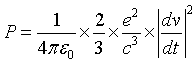(2.1) Using Eq. (2.1) we deduce the corresponding dissipated energy per unit time dE/dt (using k = 1/4πε0 and the acceleration a = dv/dt) as follow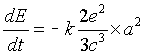(2.2) The Lamor′s formula is applied to any charged particle in motion in an electromagnetic field and it depends not on the nature of its trajectory which can be linear, circular or elliptic. In cosmology, a celestial object of mass M is accelerated in the gravitational field created by a most heavy aster of mass Mc. Let us then consider an atomic system and the Solar system. Electrons (planets) are orbiting the nucleus (the Sun) and each electron (planet) is sustained to a centripetal acceleration a. Let us move on considering the expression of the Coulomb′s force (between a nucleus of charge q and an electron of charge −e) and the gravitational force given by the 4th Newton′s laws respectively.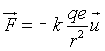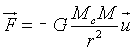These relations indicate that, the force acting on an electron (a planet) is in direct proportion to the charge of the electron (to the mass of the planet) and in inverse proportion to the square of its distance from the nucleus (from the Sun). So one can do the following analogy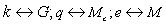. According to this analogy an electric charge is equivalent to a mass for a celestial object. We assume then, in a gravitational field created by a heavy aster of mass Mc, an accelerated astronomical system of mass M dissipated its energy per unit time dE/ dt given analogously to Eq. (2.2) by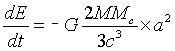(2.3) In this equation, G is the gravitational constant: G = 6.64 × 10−11 SI (it is the analogous of the electric constant k = 1/4πε0 = 9 × 109 SI as stated above). Expression of the total evaporation time of a given celestial object orbiting a gravitational center In an atomic system, each electron orbiting the nucleus is sustained to a centripetal acceleration a. In the framework of the independent particles model, each electron orbits freely the nucleus. Then, from the viewpoint of classical electrodynamics, a given electron radiates the power P given by (2.2). The loss of energy involves that the electron will lastly come across the nucleus according to classical electrodynamics previsions. In the purpose of the present study, we intend to apply Eq. (2.3) to the calculation of the evaporation time of a planet of star attracted by its center of gravitation being collapsed. Adopting the independent particles (planets or stars) model, we consider the interaction between a celestial object (planet or star) and its center of gravitation (star or galactic nucleus) ignoring the presence of the other asters orbiting the considered center. Using this approximation, we consider a moment when the celestial object engages a process of evaporation while being attracted by it center of rotation being collapsing gravitationally. The energy dissipated by the aster of mass M is then given by Eq. (2.3). For the Solar system on which we focus, it is well know that - The planetary orbit is not a circle, but an ellipse. - The Sun is not at the center but at a focal point of the elliptical orbit, - The linear speed of the planet in the orbit is constant, but the area speed is constant. - In the present work, we adopt the model of Copernicus where the eccentricities of the planetary orbits are taken as zero. In this approximation, - The planetary orbit is a circle, - The Sun is at the center of the orbit, - The speed of the planet in the orbit is constant. Let us then consider a planet of mass M orbiting initially a star of mass Mc at the distance R. In the framework of the Newtonian gravitational theory, the total energy E of such system verifies the relations (noting Ec the kinetic energy of the planet).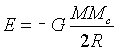(2.4)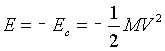(2.5) With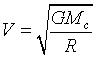(2.6) On the other hand, the centripetal acceleration is given by the wellknown expression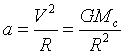(2.7) Using (2.7), Eq. (2.3) can be rewritten in the form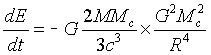(2.8) Let us derivate Eq. (2.4) with respect to time. We get(2.9) From both the Eqs. (2.8) and (2.9) we obtain then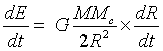(2.10) That means R2dR = − Adt (2.11) where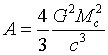Eq. (2.11) gives the relation satisfies by the planetary orbital radius during the evaporation process in the framework of the present model of destruction of an aster swallowed by its center of Mc mass collapsing gravitationally. Integrating Eq. (2.11) between the limits R0 and R(t), we obtain R30 − R3 (t) = 3A (t − t0) (2.12) In this equation, R0 denotes the orbital radius of the planet (the star) at the date t0where it starts just the evaporation process and R (t) the orbital radius of the planet (the star) at the date t during the evaporation. In the present model, the planet (the star) in a gravitational collapse event will disappear completely when the orbital radius R (t) is equal to zero. If we denote T this duration, we obtain from (2.12) R30 = 3AT. That means using the value of A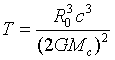(2.13) This result gives the total evaporation time of a destroyed planet or star after a gravitational collapse process of its center of rotation of mass Mc (star or galactic nucleus). Critical radius of a star collapsing gravitationally Using Eq. (2.7), we can rewrite (2.3) in the form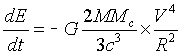(2.14) Extracting R from Eq. (2.6) and after putting the result obtained into Eq.(2.14), we get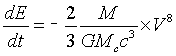(2.15) From (2.5) we pull dE/dt. So Eq. (2.15) gives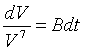(2.15) with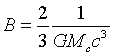Integrating (2.15) between the limits V0 and V(t), V0 = (GMc/R0)1/2, we find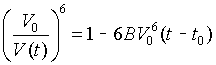(2.16) Substituting the expression of (t − t0) given by Eq.(2.12) into Eq.(2.16), we obtain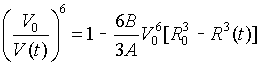(2.17) Using the values of A and B and that of V0 = (GMc/R0)1/2,, Eq.(2.17) gives the simple formula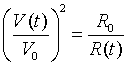(2.18) As the limit of speed is that c of light, let us put V(t) = λ×c. Eq.(2.18) gives then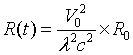(2.19) In the present model, λ2 can take only the values 1/2, 1/3, 1/4, 1/5….; as [V(t)/c]2 = λ2 < 1. During the vaporization of the planet, we assume that the star of mass Mc is simultaneously engaged in a process of gravitational collapse while attracting the planet. A particular phenomenon occurs when the evaporated planetary remaining mass ‘’touches’’ the surface of the star of R(t) radius. Let us considered this moment as a critical date tc corresponding to the star critical radius R(tc) = Rc given by [for λ = λc]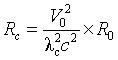(2.20) We assume that, at the date tc, the speed of the evaporated planetary mass takes a maximum value corresponding to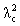= 1/2. So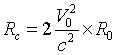(2.21) As V0 = (GMc/R0)1/2, the critical radius of the star collapsing gravitationally is expressed by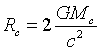(2.22) Results and Discussion Schwarzschild’s radius In the case of the Sun (Mc = MS = 2×1030 kg), the critical radius takes the value Rc = 2.95 km. This result is well-known and Eq. (2.22) corresponds then to that cosmologists call Schwarzschild radius . In the present model, when the speed of the evaporated planetary mass being attracted by a star collapsing gravitational satisfies the ratio [V(t)/c]2 > 1/2, the radius of the star becomes strictly inferior to its critical radius (2.22). The star turns into a dark hole and the gravitational collapse cannot be stopped. Subsequently, the star is reduced to a point in a very short time. Let us estimate this time in the case of the Sun (although the Sun is not enough massive to turn into a dark hole) and the Galactic nucleus (Galactic center). We assume all the planetary matter being evaporation since the distance Rc with respect to the center of a star collapsing gravitational. According to Eq. (2.12), we get using the value of the constant A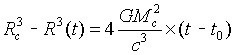(2.23) When the star disappears at the dateτ, R(t) = 0. So, we find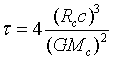(2.24) As GMc = (c2Rc)/2 according to (2.22), we obtain finally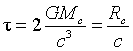(2.25) For the Sun (Mc = MS = 2×1030 kg) we find numerically τ =10 −5s. For the Galactic nucleus (Mc = MNG = 1041 kg) we find numerically = 6 days. These times could be considered as very short at the gravitational scale. Complete evaporation time of the planets of the Solar system Let us state again that, in the present model, we consider each planet as independent during the evaporation process. That means collisional interactions between planets are ignored in our calculations. Then using Eq. (2.13), one can estimate the time necessary for each planet of the Solar system to evaporate completely during a gravitational collapse of the Sun being destroyed. The results obtained are listed in Table 1. In the hypothesis of a tenth planet belonging to the Solar system, the results quoted in Table 1 point out that, the Sun collapsing gravitationally will lose all its planets when the tenth planet disappears completely at the date 1.7 × 1016 years. This result can be compared with that of Dicus et al. . In the theory of Dicus et al. , the evaporation of the planets of a solar system is described in a model invoking collisional interaction processes between two stars. Assuming a collisional interaction to occur probably at each 1015 years, Dicus et al.  explains that, after 100 collisions, all the planets of the considered star will be expelled out of their orbit, so that, about the date 100 x 1015 yr e.g. 1017 yr, all the stars of the universe will lose their planets. In the present calculations, the Sun will lose all its planets at 1016 yr (assuming that the planets are lost and destroyed). This result agrees with the previsions of Dicus et al.  considering that, the date 1017 yr concerns all the solar systems and not that of ours only. On the other hand, we can precise the probable number of collisions necessary to the expulsion of all the tenth planets in the view point of the theoretical model of Dicus et al. . Then, if we adopt the model of Dicus et al. , the time TN necessary for a star to lose all its planets after N collisions can be written in the form TN = N x 1015 yr. As for the Solar system TN = 17 × 1015 yr according to our predictions, we find then N = 17. So, about 17 collisions between the Sun and another star will be probably sufficient to evict all the planetary envelope of the Solar system. The present agreement points out that, the model adopted in the present work ignoring collisional interaction between planets and between planets and stars and the model of Dicus et al.  can be used to evaluate the duration of the destruction of a celestial object by assuming that at the loss of the celestial object means its destruction or evaporation. Evaporation time of the sun We consider here a model where the mass MS of the Sun is being engulfed by the nucleus of the Galaxy collapsing gravitationally. As the Sun orbits the galactic nucleus at the average distance 27 000 light years (2.55 × 1020 m) and considering that the Galaxy nucleus mass is equal approximately to 10 41 kg, Eq. (2.13) gives for the Sun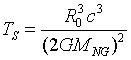(2.26) Numerically, we obtain Ts = 2.52 × 1024 s. In year units, we get (using for time conversion: 1 year = 365.25 days), Ts = 7.97 × 1016 yr. This result points out that, after losing all its planets at the approximate date 2 × 1016 yr (date of disappearing of fictitious tenth planet of the Solar system), the Sun will not be completely destroyed as Ts ≈ 8 × 1016 yr > 2 × 1016 yr. This result is very satisfactory as according to Reeves , after the evaporation of the external planets of the Solar system, Jupiter, Saturn, Uranus, Neptune and Pluto (considered here as a planet even if astronomers had fixed the numbers of the planets of the Solar System to eight  the Sun at the point of death will not disappears completely. A kind of denuded nucleus (a white dwarf like that orbiting Sirius) will remain in the place of the Sun gravitationally collapsed. Limit of the solar system Let us now move on determining the probably limit of the Solar system. If we agree with Reeves  that the Sun will not disappears after losing all its planets, this assumption means that, it is impossible to a planet belonging to the Solar system to disappear at the same time Ts = 7.97 × 1016 yr that the Sun. In addition let us considerer a fictitious planet orbiting the Sun at the limit Rlim = ρlim. Let us then compel this planet to disappear at the same time that the Sun. This involves using (2.13)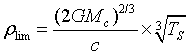(2.27) Numerically ρlim= 2. 44 ×1010 km = 163 AU In July 2005, 2003UB313 has been discovered as the most distant object yet seen in the Solar system, 97 times (97 AU) further from the Sun than the Earth is . The present prediction ρlim= 163 AU shows that 2003UB313 is indeed a celestial object belonging to the Solar system. But, it is important to underline that, the limit 163 AU is calculated in the hypothesis of a fictitious planet evaporating at the same time that the Sun collapsing gravitationally. As astronomical previsions  state that the Sun at the point of death will not disappears completely after losing all its planets, the limit of the Solar system may be strictly inferior to 163 AU (value obtained in the basis of R0 = 27 000 light-years and MS = 2 × 1030 kg for the Sun). This points out that, there is not a celestial object orbiting the Sun beyond 160 AU (value which might be considered as the limit of the Solar system). Before closing the present section, it is important to discuss about how would be the problem if an elliptical trajectory of the electron is considered and until where the Larmor’s formula is valid? As the orbit of a planet is an ellipse with the Sun at one of the two focal points, in the viewpoint of mathematics, in polar coordinates (r, θ) an ellipse can be represented by the formula: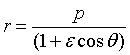(2.28) In this expression, p is the semi-latus rectum, ε denotes the eccentricity of the ellipse, r represents the distance from the Sun to the planet, and θ is the angle to the planet's current position from its closest approach, as seen from the Sun. In addition, the semi-major axis a and semi-minor axis b satisfy the equation b2 = a2 (1 − ε)2. For a circular orbit (ε = 0), r = a= b = p. Taking into account the elliptical orbit, the speed and the acceleration of a given planet are obtained differentiating the position vector. So, the expressions of the speed and the acceleration of a planet in polar coordinates will differ notably to the simple formulas (2.6) and (2.7). As a result, the evaporation time given by Eq. (13) can be corrected considering and elliptical orbit. On the viewpoint of mathematics, an analytical expression could be obtained otherwise numerical calculations might be required. Study is in such direction. In addition, in the recent work of Tаenvаt , the third Kepler’ law is corrected as T2/a2.9977948. So Eq. (2.12) will be is the form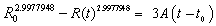.In this regard, one may substitute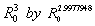. In the particular case of the Earth, R0 = 149.5 × 109 m. We find for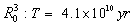for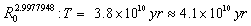. This particular result indicates that, the slight modification of the third Kepler’s law in the form (T2)/(a2.9977948) will not affect significantly the value of the evaporation time T because 2.9977948 ≈ 3.00. Subsequently, the values of T quoted in Table 1 obtained with respect to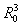can be used bringing in mind that these values are just an estimation (not the exact values) of the evaporation times of a given planet of the Solar System. Besides, the Larmor’s formula (2.1) is used to calculate in electrodynamics, the total power radiated by a nonrelativistic point charge as it accelerates or decelerates. For speeds that are small relative to the velocity of light, the total power radiated is given by the (2.1). For a planetary system like the Solar system where classical mechanics is valid, Eq. (2.3) giving the power dissipated by an aster of mass M accelerated in a gravitational field of an aster of mass Mc is applicable. One may just identify the nature of the trajectory. In the present work, circular trajectory is considered as a first approximation in the calculation of the evaporation time of the planets of Solar system. Summary and Conclusion With a simple analogous model between an atomic system and the Solar system, an equation giving the energy dissipated by an aster accelerated in a gravitational field (EDGF) is presented in this paper. EDGF has permitted to find again the Schwarzschild radius and to estimate the total evaporation times of the planets of the Solar system and that of the Sun itself. As far as we know, the present study gives the first estimations of the evaporation times of the planets of the Solar system in a model where they are engulfed by the Sun collapsing gravitationally. Overall, the results obtained are in good agreement with very scarce astronomical previsions. EDGF has also permitted to estimate the limit of the Solar system, an important criterion for fixing the mean condition to be satisfied by a planet belonging to the Solar system. This point is extremely important as it is obvious that the Solar system is not infinite. When classical mechanics is valid, the present EDGF may be used to calculate the total power radiated by a non-relativistic aster accelerated or decelerated in a gravitational field. Remark “In the year 1964, a group of five comets have been discovered and their aphelia are about 50, 800, 000, 000 km of the Sun. This localizes them around the orbit of Pluto. It’s interesting to notice that, in these conditions, for sixteen identified comets, the aphelion is situated to more than 11, 000, 000, 000 kilometers of the Sun. As most of things relative to the Solar System, the Bode’s low is especially an amusing curiosity. But, the progression let well supposed that the sixteen identified comets which the aphelia is at 11, 000, 000, 000 kilometers of the Sun are dominated by a tenth planet who follows an orbit with an average distance to the Sun equals to this aphelia” . Even if the preceding astronomical observations date on 1964, comparison with our results 11, 302, 000, 000 kilometers indicates a good agreement. This points out that, the tenth planet (or a tenth celestial object like Pluto) may be researched among the sixteen identified comets which the aphelia are at more than 11, 000, 000, 000 kilometers of the Sun even if the Prague (IAU0603 2006) statement  considered the Solar System as composed of eight planets. An interesting challenge is then opened by reviewing the Prague statement as it has been announced in 2005 that astronomers discovered new planet, larger and more distant than Pluto . References Schröder KP (2008) Distant future of the Sun and Earth revisited, Monthly Notices of the Royal Astronomical Society 386: 155–163. Sackmann I (1993) Our Sun. III. Present and Future, The Astrophysical Journal 418: 457–468. Nola Taylor Redd (2016) Red Giant Stars: Fact, Definition & the Future of the Sun.Space.com. Retrieved 20 February. Schröder KP, Connon Smith R (2008) Distant future of the Sun and Earth revisited". Monthly Notices of the Royal Astronomical Society 386: 155–163. Futurism (2015) How Will Our Solar System (and our planet) Die? Ricon P (2006) Pluto vote 'hijacked' in revolt, Science reporter, BBC News. Reeves H (1981) Patience dansl’azure, l’évolutioncosmique, Editions du Seuil, Paris 6: 136-137. Dicus D, Letaw J, Teplitz D, Teplitz V (1988) Le futur de l’Univers, Bibliothèque pour la Science, Edition Belin, Paris pp-216. Boulanger O (2004) SEDNA : la dixièmeplanète de notresystèmesolaire ? Sciences Actualités. Martin P (2005) Astronomers discover new planet, larger and more distant than Pluto International Committee of the Fourth International (ICFI), World Socialist Web Site. Hecht J (2005) Tenth planet discovered in outer solar system, New Scientist Space. Jackson JD (2001) Electrodynamiqueclassique, Dunod, Paris pp: 697. Robert LG (1975) Le SystèmeSolaire, BIBLIOTHEQES LAFFONT DES GRANDS. Tаenvаt MM (2015) Power Regression as an Example of the Third Law of Hotels in Paris: Planets J Astrophys Aerospace Technol 3: 124. Nourse EA (1964) Rendez-vous avec les planètes, Nouveaux horizons, Paris p:165. International Astronomical Union (IAU) (2006) Result of the IAU Resolution votes IAU0603 Prague.

#### Tables and Figures at a glanceTable 1
Select your language of interest to view the total content in your interested language

### Article Usage

• Total views: 8637
• [From(publication date):
December-2016 - Jan 18, 2020]
• Breakdown by view type
• HTML page views : 8537Can't read the image? click here to refresh